# Megamos chip

Input is marked red and output green.

Inverted output is marked turquoise, clock yellow and reset is gray/black.

Where no port names are given, the inputs are named A,B,... from left to right.

Flip Flop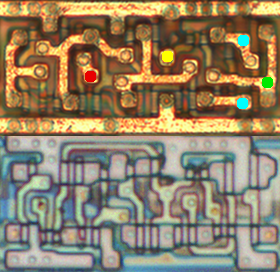(.xcf) 01: FlipFlop1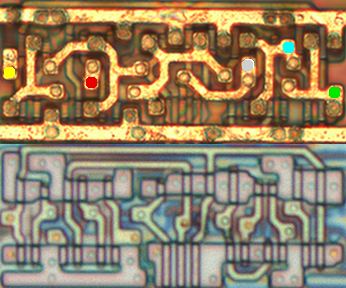(.xcf) 02: FlipFlop(.xcf) 03: FlipFlop(.xcf) 66: FlipFlop

Latch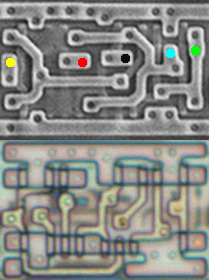(.xcf) 35: Latch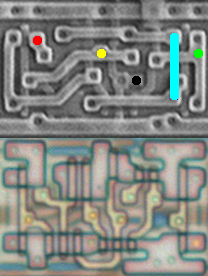(.xcf) 61: Latch

XOR/XNOR(.xcf) 10: XOR Y = (A xor B)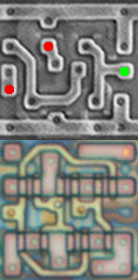(.xcf) 24: XOR Y = (A xor B)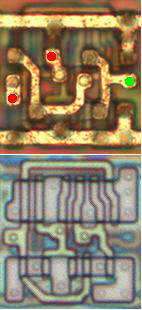(.xcf) 30: XOR Y = (A xor B)(.xcf) 06: XNOR Y = ~(A xor B)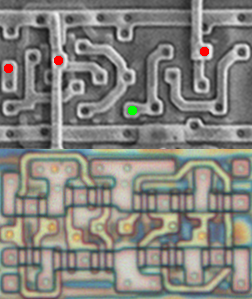(.xcf) 13: XNOR Y = ~(A xor B)

AND/NAND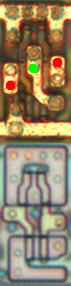(.xcf) 10: NAND Y = ~(A and B)(.xcf) 14: NAND Y = ~(A and B and C and D)(.xcf) 20: NAND Y = ~(A and B and C)(.xcf) 21: NAND Y = ~(A and B and C)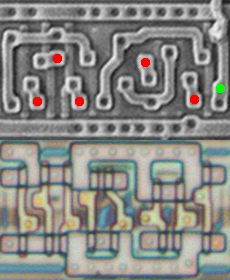(.xcf) 54: NAND Y = ~(A and B and C and D and E)(.xcf) 39: AND Y = (A and B)(.xcf) 45: AND Y = (A and B and C)

OR/NOR(.xcf) 19: NOR Y = ~(A or B)(.xcf) 38: 2-NOR Y = ~(A or B)(.xcf) 44: NOR Y = ~(A or B or C)(.xcf) 65: NOR Y = ~(A or B)(.xcf) 48: OR Y = (A or B or C)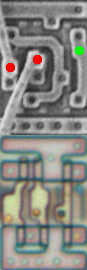(.xcf) 63: OR Y = (A or B)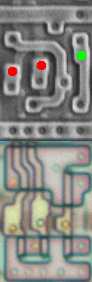(.xcf) 70: OR Y = (A or B)

Buffer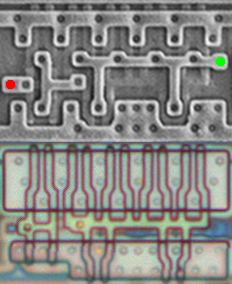(.xcf) 04: Buffer Y = A(.xcf) 18: Buffer Y = A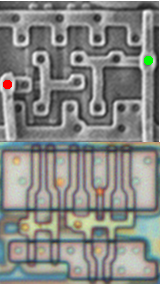(.xcf) 23: Buffer Y = A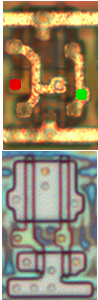(.xcf) 25: Buffer Y = A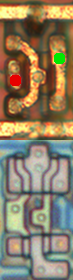(.xcf) 46: Buffer Y = A

Inverter(.xcf) 31: Inverter Y = ~A(.xcf) 32: Inverter Y = ~A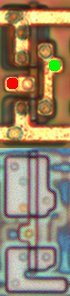(.xcf) 40: Inverter Y = ~A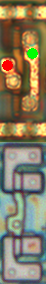(.xcf) 50: Inverter Y = ~A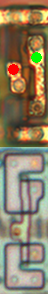(.xcf) 51: Inverter Y = ~A

Mux(.xcf) 16: Mux Y =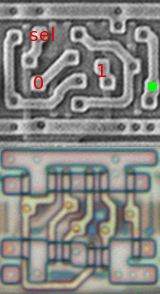(.xcf) 37: Mux Y =(.xcf) 47: Mux Y =

misc(.xcf) Y = ~((A and B) or (B and C) or (A and C))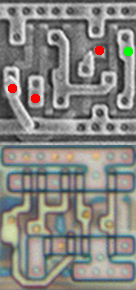(.xcf) Y = (B or (A and C))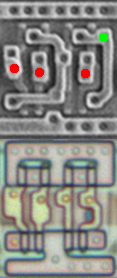(.xcf) Y = ~(~(A and B) and C)(.xcf) Y = ~((A and D) or B or C)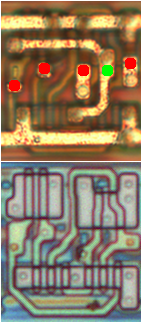(.xcf) Y = ~((A or B) and C and D)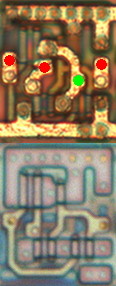(.xcf) Y = ~((A or B) and C)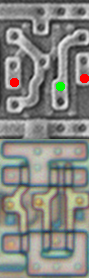(.xcf) Y = A and ~B(.xcf) Y = (A or B) and (~C or ~D)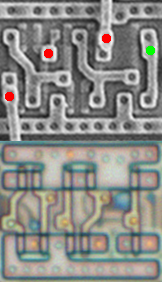(.xcf) Y = ~A and ~B and C(.xcf) Y = ~((A and C) or B)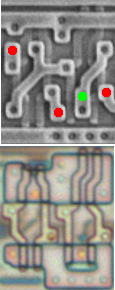(.xcf) Y = A and B and ~C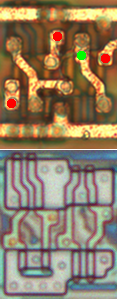(.xcf) Y = A or B or ~C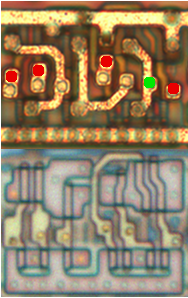(.xcf) Y = A or B or ~(C or D)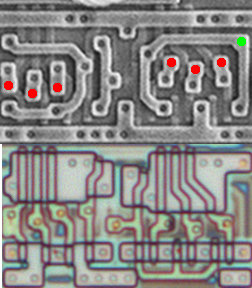(.xcf) Y = ~(A or B or C) or ~(D or E or F)(.xcf) Y = ~(A or B) or C or ~(D or E)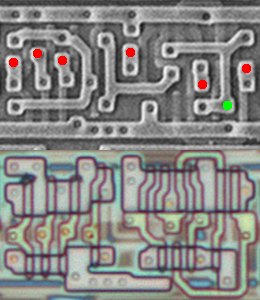(.xcf) Y = A and B and C and ~(D and E and F)